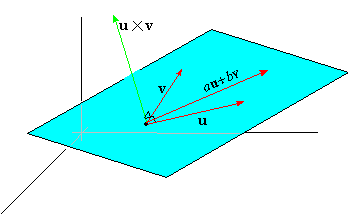### Part 4: The Span of Two Non-parallel Vectors

A linear combination of non-parallel vectors u and v is a vector of the form
 au+bv
where a and b are numbers. The set of all linear combinations of 2 non-parallel vectors u and v is called the span of u and v.Moreover, if u and v are parallel to given plane P, then the plane P is said to be spanned by u and v.

EXAMPLE 7    Find the equation of the plane through the point P1( 0,0,0) spanned by the vectors u = á1,2,1 ñ and v = á 3,1,-2 ñ

Solution: The normal to the plane is n = u×v, which is
 n = u×v = á 1,2,1 ñ × á 3,1,-2 ñ = á -5,5,-5 ñ
Definition 4.1 then implies that the equation of the plane is
 -5( x-0) +5( y-0) -5( z-0) = 0
Solving for z then produces the functional form z = y-x.

Finally, since r×( u×v) is orthogonal to u×v, the vector r×( u×v) must be in the span of u and v. That is, there must be scalars a and b such that r×(u×v) = au+bv. Also, since r×( u×v) is orthogonal to r, we have
 r·( au+bv) = 0       or       ar·u = -br·v
Indeed, it is straightforward to show that  a = r·v and b = -r·u , thus leading to the triple vector product identity:
 r×( u×v) = ( r·v)  u-( r·u)  v
(4)
Exercises 41-44 will provide additional insights into the interpretation and application of (4).

EXAMPLE 8    Verify (4) for the vectors
 u = á 1,2,0 ñ ,  v = á1,1,0 ñ ,  and  r = á 3,2,1 ñ

Solution: Since u×v = á0,0,-1 ñ , the left side of (4) is
 r×( u×v) = á3,2,1 ñ × á 0,0,-1 ñ = á-2,3,0 ñ
Notice that u, v, and r×( u×v) are parallel to the xy-plane.  The right side of (4) is
 ( r·v)  u-( r·u) v
 =
 ( á 3,2,1 ñ · á1,1,0 ñ )  u-( á3,2,1 ñ · á 1,2,0 ñ )  v
 =
 5u-7v
 =
 5 á 1,2,0 ñ -7 á 1,1,0 ñ
 =
 á 5-7,10-7,0 ñ
 =
 á -2,3,0 ñ